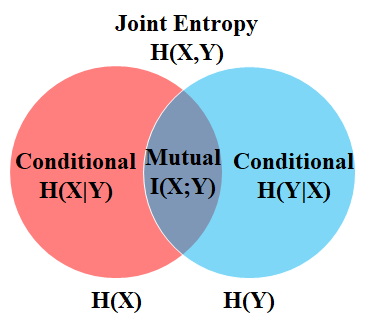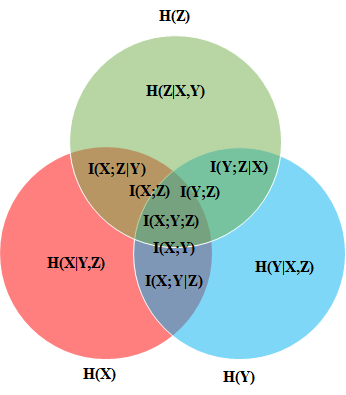• 首先，我们要测量服务之间语义关联静电力理论中基于库仑力公式电子元件。 为此，通过对服务调用历史日志进行统计计算，我们提供了一种基于PageRank方法来度量服务组件重要性，并定义它们之间距离度量。...
• 论文研究-基于灰色关联系数和D-S证据理论的区间数投资决策方法.pdf, 主要研究投资方案的指标值为区间数时的投资方案选择问题.定义了效益型和成本型指标下的理想属性区间...
• 这个还是让我们从会话保持的定义谈起。做一个形象的比喻：会话保持可以看做一串珍珠，那么我们把每一个珍珠拆下来放到不同的盒子里面进行保存处理，之后在拿出来穿成串，也就是一个分配的概念。那么，学术上是怎么...

F5是负载均衡器产品中的知名者，相信很多人都不会不知道的。那么，它是如何进行会话保持的呢？这个还是让我们从会话保持的定义谈起。做一个形象的比喻：会话保持可以看做一串珍珠，那么我们把每一个珍珠拆下来放到不同的盒子里面进行保存处理，之后在拿出来穿成串，也就是一个分配的概念。那么，学术上是怎么描述的呢？还是让我们从下文了解吧。

1.什么是会话保持?

在大多数电子商务的应用系统或者需要进行用户身份认证的在线系统中,一个客户与服务器经常经过好几次的交互过程才能完成一笔交易或者是一个请求的完成｡由于这几次交互过程是密切相关的,服务器在进行这些交互过程的某一个交互步骤时,往往需要了解上一次交互过程的处理结果,或者上几步的交互过程结果,服务器进行下一步操作时需要这就要求所有这些相关的交互过程都由一台服务器完成,而不能被负载均衡器分散到不同的服务器上｡

而这一系列的相关的交互过程可能是由客户到服务器的一个连接的多次会话完成,也可能是在客户与服务器之间的多个不同连接里的多次会话完成｡不同连接的多次会话,最典型的例子就是基于http的访问,一个客户完成一笔交易可能需多次点击,而一个新的点击产生的请求,可能会重用上一次点击建立起来的连接,也可能是一个新建的连接｡

会话保持就是指在负载均衡器上有这么一种机制,可以识别做客户与服务器之间交互过程的关连性,在作负载均衡的同时,还保证一系列相关连的访问请求会保持分配到一台服务器上｡

2.F5支持什么样的会话保持方法?

F5 BigIP支持多种的会话保持方法,其中包括:简单会话保持(源地址会话保持)､HTTP Header的会话保持,基于SSL Session ID的会话保持,I-Rules会话保持以及基于 HTTP Cookie的会话保持,此外还有基于SIP ID以及Cache设备的会话保持等,但常用的是简单会话保持,HTTP Header的会话保持以及 HTTP Cookie会话保持以及基于I-Rules的会话保持｡

◆简单会话保持

简单会话保持也被称为基于源地址的会话保持,是指负载均衡器在作负载均衡时是根据访问请求的源地址作为判断关连会话的依据｡对来自同一IP地址的所有访问请求在作负载均时都会被保持到一台服务器上去｡在BIGIP设备上可以为“同一IP地址"通过网络掩码进行区分,比如可以通过对IP地址 192.168.1.1进行255.255.255.0的网络掩码,这样只要是来自于192.168.1.0/24这个网段的流量BIGIP都可以认为他们是来自于同一个用户,这样就将把来自于192.168.1.0/24网段的流量会话保持到特定的一台服务器上｡

简单会话保持里另外一个很重要的参数就是连接超时值,BIGIP会为每一个进行会话保持的会话设定一个时间值,当一个会话上一次完成到这个会话下次再来之前的间隔如果小于这个超时值,BIGIP将会将新的连接进行会话保持,但如果这个间隔大于该超时值,BIGIP将会将新来的连接认为是新的会话然后进行负载平衡｡

基于原地址的会话保持实现起来简单,只需要根据数据包三､四层的信息就可以实现,效率也比较高｡存在的问题就在于当多个客户是通过代理或地址转换的方式来访问服务器时,由于都分配到同一台服务器上,会导致服务器之间的负载严重失衡｡另外一种情况上客户机数量很少,但每个客户机都会产生多个并发访问,对这些必发访问也要求通过负载均衡器分配到多个服器上,这时基于客户端源地址的会话保持方法也会导致负载均衡失效｡

展开全文会话保持 负载均衡
• 一个事务数据库中的关联规则挖掘可以描述如下：设 I = { i1,2,i3...,im}是一个项目集合 ，事务数据库D ={t1,t2,...tn}是由一系列具有唯一标识TID事务组成。每一个事务ti(i =1,2,...,n)都对应I上一个子集。 2. ...
文章目录一、基本概念与解决方法1. 事务数据库2. 定义3. 解决方法二、经典的频繁项目集生成算法分析1. 项目集空间理论2. Apriori算法1. 算法的基本思想2.步骤：3. 核心步骤4. 伪代码5. 例题3. 关联规则的生成1. 步骤2. 例题3. 关联规则优化
一、基本概念与解决方法
1. 事务数据库
交易数据库又称事务数据库，尽管它们英文名词一样，但事务数据库更具有普遍性。


一个事务数据库中的关联规则挖掘可以描述如下：设 I = { i1,2,i3...,im}是一个项目集合 ，事务数据库D ={t1,t2,...tn}是由一系列具有唯一标识的TID事务组成。每一个事务ti(i =1,2,...,n)都对应I上的一个子集。2. 定义

定义3.1：支持度（集合在 I 中出现的次数）定义3.2：频繁项目集
对于项目集I，在事务数据库D中所有满足用户指定的最小支持度(Minsupport) 的项目集，即不小于Minsupport的I的非空子集，称为频繁项目集（Frequest Itemsets）或大项目集定义3.3：可信度（比例）定义3.4：强关联规则

D在I上满足最小支持度和最小置信度的关联规则称为强关联规则。
通常说的关联规则一般是指强关联规则
关联规则挖掘定义：给定一个事务数据库，通过用户指定的最小支持度和最小置信度来寻找强关联规则的过程。3. 解决方法
关联规则挖掘可以划分为两个子问题

发现频繁项目集： 通过用户给定的最小支持度，寻找所有的频繁项目集，即满足支持度Support不小于Minsupport的所有项目子集。发现所有频繁项目子集是形成关联规则的基础。
生成关联规则： 通过用户给定的最小可信度，在每个最大频繁项目子集中寻找置信度不小于MInconfidence的关联规则。
发现频繁项目子集是近年来的研究重点

二、经典的频繁项目集生成算法分析
1. 项目集空间理论
频繁项目集的子集仍是频繁项目集；非频繁项目集的超集仍是非频繁项目集。2. Apriori算法
1. 算法的基本思想
基于频繁项目集性质的先验知识，使用由下到上逐层搜索的迭代方法，k项集用于搜索K+1项集。

2.步骤：

首先扫描数据库，统计每一个项发生的数目，找出满足最小支持度的项， 找出频繁1项集，记作L1
然后，**基于L1找出频繁2项集的集合L2，基于L2找出频繁3项集的集合L3，**如此下去，直到不能找到频繁K项集LK。找每一个Lk需要一次数据库全扫描。

3. 核心步骤
Apriori算法的核心由连接步和剪枝步组成。

连接步：  为找频繁项集Lk（k  > 2），先通过将Lk-1于自身连接产生 候选K项集Ck。剪枝步： 由候选K项集的集合Ck产生频繁项集Lk，Ck是Lk的超集。从Ck中确定Lk。为了压缩Ck，可以利用先验知识：任何非频繁的（k-1）项集都不是频繁K项集的子集。4. 伪代码5. 例题

例题1解释： 我们的数据集D有4条记录，分别是134,235,1235和25。现在我们用Apriori算法来寻找频繁k项集，最小支持度设置为50%。首先我们生成候选频繁1项集，包括我们所有的5个数据并计算5个数据的支持度，计算完毕后我们进行剪枝，数据4由于支持度只有25%被剪掉。我们最终的频繁1项集为1235，现在我们链接生成候选频繁2项集，包括12,13,15,23,25,35共6组。此时我们的第一轮迭代结束。
进入第二轮迭代，我们扫描数据集计算候选频繁2项集的支持度，接着进行剪枝，由于12和15的支持度只有25%而被筛除，得到真正的频繁2项集，包括13,23,25,35。现在我们链接生成候选频繁3项集,123, 125，135和235共4组，这部分图中没有画出。通过计算候选频繁3项集的支持度，我们发现123,125和135的支持度均为25%，因此接着被剪枝，最终得到的真正频繁3项集为235一组。由于此时我们无法再进行数据连接，进而得到候选频繁4项集，最终的结果即为频繁3三项集235。

例题23. 关联规则的生成
1. 步骤
得到了所有的频繁项目集后，可以按照下面的步骤生成关联规则。

对于每一个频繁项目集Lk，生成其所有的非空子集。
对于Lk的每一个非空子集x，计算Cnference(x -> (Lk - x))
如果Conference(x - > (Lk - x))  = support(Lk) / support(x) >=  minconfidence ，那么x -> (Lk - x) 成立上述算法的核心是genrules递归过程，它实现一个频繁项目集中所有强关联规则的生成。2. 例题3. 关联规则优化
设频繁项目集I，项目集 x 属于 I，x1为x的一个子集。如果 x ->(Lk - x)不是强关联规则，那么 x1->(Lk-x1) 一定不是强关联规则。设项目集X，X1是X的一个子集，如果规则Y-> X是强规则，那么规则 Y -> X1 一定是强规则。展开全文Apriori
• 众所周知，但有时被忽略的是，对倾斜参数（最主要是S和T参数）的约束通常仅适用于称为通用理论的一类特殊的新物理场景。 在有效场论（EFT）框架中，除非在EFT上施加了限制以描述通用理论，否则倾斜参数不应在特定的...
• 熵（Entropy）的理论知识 定义 在信息论中，熵被定义为随机变量平均不确定度度量。也是平均意义上描述随机变量所需信息量度量。 设XXX是一个离散型随机变量，其字母表（即概率论中取值空间）为χ\chiχ。...
目录标题熵（Entropy）的理论知识定义例子1. 均匀分布2. 非均匀分布联合熵（joint entropy）条件熵（conditional entropy）相对熵（relative entropy）或(Kullback-Leibler)KL散度交叉熵（cross entropy）分类问题损失函数互信息（mutual entropy）信息增益（information gain）熵之间的关系链式法则熵的性质多变量分布熵条件互信息（conditional mutual information）条件相对熵（conditional relative entropy）多变量链式法则熵的链式法则互信息的链式法则相对熵的链式法则参考
传送门：信息熵在tensorflow2.*中实现参考博文
熵（Entropy）的理论知识
定义
在信息论中，熵被定义为随机变量的平均不确定度的度量。也是平均意义上描述随机变量所需的信息量的度量。
设$X$是一个离散型随机变量，其字母表（即概率论中的取值空间）为$\chi$。概率密度函数$p(x)=Pr(X=x), x\in\chi$， 则一个离散随机变量$X$的熵$H(X)$定义为
$H(X)=-\sum p(x)\log_{2} p(x)$
表达式中对数函数以2为底，熵的量纲为比特。在平均意义下，熵是为了描述随机变量$X$所需的比特数。
数学期望表达式
如果$X \sim p(x)$，随机变量$g(x)$的期望值可记作：
$E_{p} g(x) =\sum_{x\in\chi}g(x)p(x)$
当$g(x)=log \frac{1}{p(X)}$时， $H(X)$可以表示位随机变量$log \frac{1}{p(X)}$的期望值，即
$H(X)=E_{p} log \frac{1}{p(X)}$
例子
1. 均匀分布
一个服从均匀分布且有32种可能结果的随机变量，需要用多长的字符串描述这个随机变量？
首先易得使用二进制需要$2^5=32$，即5字节的长度。
由熵公式可得，$H(X)=-\sum_{x=1}^{32} p(x)log(px)=-\sum_{x=1}^{32} \frac{1}{32}log\frac{1}{32}=5$比特，恰好等于描述32的字节长度
2. 非均匀分布
书中举了一个实际的例子，假定一个有8匹马的比赛，8匹马的获胜概率分别位$(\frac{1}{4}, \frac{1}{8}, \frac{1}{16}, \frac{1}{32}, \frac{1}{64}, \frac{1}{64}, \frac{1}{64},\frac{1}{64})$。现在需要在马赛比赛结束的第一时刻把消息传播出去，并使用传播所需的信息最小。
有两种策略：

对所有参赛的马按照相同策略进行编码
此时8匹马需要使用$2^3=8$，即使用常规的二进制编码方式从000到111进行编码。因此，对任何一匹马都选哟3字节长度。
可能性较大的马使用较短的编码
这样对应8匹马分别使用：0，10，110，1110， 111100，111101，111110，111111的一组二元字符串进行表示的话。对每一匹马的编码长度乘以对应概率值求和，刚好等于2，小于方法一中的3字节长度。

策略一中为什么不使用一位和两位的字符？

同时，由求熵公式可得
$H(X)=- \frac{1}{2}log\frac{1}{2}- \frac{1}{4}log\frac{1}{4}- \frac{1}{8}log\frac{1}{8}- \frac{1}{16}log\frac{1}{16}- \frac{1}{32}log\frac{1}{32}- \\\frac{1}{64}log\frac{1}{64}- \frac{1}{64}log\frac{1}{64}- \frac{1}{64}log\frac{1}{64}- \frac{1}{64}log\frac{1}{64}=2$
可见第二种方式得到的平均比特数正好等于熵
如果大家和我一样还不太理解可以参看信息熵和计算公式介绍视频
关键点需要理解信息和熵的定义

信息
熵

描述一个随机事件所需的字节数
随机事件不确定性的度量（单位字节）

获取有效信息，消除随机事件熵减少
对随机事件进行描述所需要的编码长度

联合熵（joint entropy）
对于服从联合分布为$p(x,y)$的一对离散随机变量$(X,Y)$，其联合熵$H(X,Y)$定义为
$H(X,Y)=-\sum_{x\in\chi}\sum_{y\in y}p(x,y)logp(x,y)$
亦可以表示为
$H(X,Y)=-Elogp(x,y)$
条件熵（conditional entropy）
已知随机变量X的条件下，随机变量 Y 的不确定性
\begin{aligned} H(Y/X)&=\sum_{x \in \chi}p(x)H(Y|X=x)\\&=-\sum_{x \in \chi}p(x)\sum_{y \in Y}p(y|x)logp(y|x)\\&=-\sum_{x \in \chi}\sum_{y \in Y}p(x,y)logp(y|x)\\&=-Elogp(Y|X) \end{aligned}
相对熵（relative entropy）或(Kullback-Leibler)KL散度
两个随机分布之间距离的度量。
例如，已知随机变量的真实分布为$p$，可以构造平均描述长度为$H(p)$的编码。但是，如果使用针对分布$q$的编码，那么在平均意义上就需要$H(p)+D(p||q)$比特的编码来描述这个随机变量

q的熵一定大于p的意思？
$D(p||q)=\sum_{x \in \chi}p(x)log \frac{p(x)}{q(x)}=E_{p}log \frac{p(x)}{q(x)}$

交叉熵（cross entropy）
两个概率分布间的差异性信息
在深度学习中最常使用 交叉熵作为损伤函数
$H(p,q)=-\sum_{x \in \chi}p(x)log(q(x))$
由相对熵的表达式
\begin{aligned} D(p||q)&=\sum_{x \in \chi}p(x)log \frac{p(x)}{q(x)}\\&=\sum_{x \in \chi}p(x)log(p(x))-\sum_{x \in \chi}p(x)log(q(x))\\&=-H(p(X))+H(p,q) \end{aligned}
可得，交叉熵$H(p,q)$恰好等于$H(p)+D(p||q)$。当$H(p)=0$时，相对熵就等于相对熵。即两者都可以表示两个随机分布的差异性，交叉熵数值包含一个真实（参考）随机分布p的熵
分类问题损失函数
对于$Y_{true}$由于进行One-hot编码后是一个001000的分布，故$H(p(X))$等于0，即$D(p||q)=H(p,q)$。损失函数$H(p,q)$减少的意义是$Y_{true}$与$Y_{pred}$分布之间的差异性逐渐减少，或距离越来越小，或采用相同的编码方式不需要额外的信息量进行描述。
互信息（mutual entropy）
一个随机变量包含另一个随机变量信息量的度量
给定另一随机变量条件下，原随机变量不确定度的缩减量
互信息$I(X;Y)$等于联合概率密度函数$p(x,y)$和边界概率密度函数$p(x)p(y)$乘积之间的相对熵
\begin{aligned} I(X;Y)&=\sum_{x \in \chi} \sum_{y \in Y}p(x,y)log\frac{p(x,y)}{p(x)p(y)}\\&=D((p(x,y)||p(x)p(y)))\\&=E_{p(x,y)}log\frac{p(X,Y)}{p(X)p(Y)} \end{aligned}
信息增益（information gain）
表达式与互信息相同，熵减去条件熵。但实际表达意义存在一定差别。
$IG(X;Y)=H(X)-H(X|Y)$
互信息中Y表示事件，信息增益Y表示分类方式
互信息里面的Y，用$H(Y)$表示，可以通过统计测量概率，并用信息熵公式计算。
但是增益里面的Y，由于是一种分类方式，它的熵要是直接计算，信息论里面没有介绍。
信息增益是描述前后两种不同状态的信息熵变化，即确定性的增加量，分类(决策树)本质就是将一个系统中各种元素之间的分类关系（X,Y,Z,…）确定下来。H(X)表示分类前的熵，H(X|Y)表示分类后的熵
熵之间的关系
链式法则
$H(X,Y)=H(X)+H(Y|X)$
联合熵等于已知条件X的熵加已知X的条件熵。
联合熵与条件熵的差等于已知条件的熵值
$I(X;Y)=H(X)-H(X|Y)=H(Y)-H(Y|X)$
$I(X;Y)=H(X)-H(X|Y)$表示在给出Y信息的条件下，X的不确定度的缩减量
将链式法则$H(X|Y)=H(X,Y)-H(Y)$代入可得
$I(X;Y)=H(X)+H(Y)-H(Y,X)$
互信息等于各自熵的和减去联合熵
至此所有两个随机变量之间的熵的关系可由下图表示出来熵的性质

$H(X)\geq0$
$H_{b}(x)=(log_{b}a)H_{a}(X)$
$H(X|Y)\le H(X)$ 条件作用使熵减少
$H(X_1,X_2,...,X_n)\le\sum_{i=1}^{n}H(X_i)$
$H(X)\le log|\chi|$
$H(p)$关于p是凹函数

多变量分布熵
条件互信息（conditional mutual information）
随机变量X和Y在给定随机变量Z时的条件互信息（conditional mutual information）
\begin{aligned} I(X;Y|Z)&=H(X|Z)-H(X|Y,Z)\\&=E_{p(x,y,z)}log\frac{p(X,Y|Z)}{p(X|Z)p(Y|Z)} \end{aligned}
条件相对熵（conditional relative entropy）
\begin{aligned} D(p(y|x)||q(y|x))&=\sum_{x}p(x)\sum_{y}p(y|x)log\frac{p(y|x)}{q(y|x)}\\&=E_{p(x,y)}log\frac{p(Y|X)}{q(Y|X)} \end{aligned}多变量链式法则
熵的链式法则
$H(X,Y)=H(X)+H(Y|X)\\ H(X,Y,Z)=H(X)+H(Y|X)+H(Z|X,Y)=H(X)+H(Y,Z|X)$
一般式：
$H(X_1,X_2,...,X_n)=\sum_{i=1}^{n}H(X_i|X_{i-1},...,X_1)$
互信息的链式法则
$I(X_1,X_2,...,X_n;Y)=\sum_{i=1}^{n}I(X_i;Y|X_{i-1},...,X_1)$
相对熵的链式法则
一对随机变量的两个联合分布之间的相对熵可以展开为相对熵和条件相对熵之和
$D(p(x,y)||q(x,y))=D(p(x)||q(x))+D(p(y|x)||q(y|x))$
参考
《Elements of information Theory》


展开全文机器学习 深度学习
• 如果多个文件使用同一个变量，就必须把声明和定义分开：变量的定义只能出现在一个文件中，而使用到它的文件必须对其声明。（不可重复定义，但可以多次声明） 如何实现：extern可声明但不定义，如果对extern进行显式...
C++理论梳理2——声明&定义（extern和文件间数据共享）
C++支持各个文件独立编译，最后链接。文件之间数据的通信就涉及”一次定义&多次声明“

声明使得名字为程序所知，定义负责创建与名字关联的实体。
如果多个文件使用同一个变量，就必须把声明和定义分开：变量的定义只能出现在一个文件中，而使用到它的文件必须对其声明。（不可重复定义，但可以多次声明）
如何实现：extern可声明但不定义，如果对extern进行显式初始化则会抵消extern的作用：变成定义。

int i;		//声明并定义
extern int i;	//声明
extern int i=10; 		//定义



展开全文c++
• 在格蕴涵代数关联滤子研究成果的基础上，将直觉模糊集理论与格蕴涵代数的关联滤子的结构及性质相结合，给出了格蕴涵代数中的直觉模糊关联滤子的定义，讨论了格蕴涵代数中直觉模糊滤子和直觉模糊关联滤子之间的关系，...
• 1、定义 设有序列，则成映射为序列x到序列y数据变换。 （1） f 是初值化变换。 （2） f 是均值化变换。 （3） f 是百分比变换 （4） f 是倍数变换 （5） f 是归一化变换其中x0>0一个数值 （6） f 是极差最大...
• 摘要:为了解决经典粗糙集理论无法处理不精确分类以及连续值属性离散化造成信息损失等问题,本文提出了基本灰色关联变经度粗糙集模型 *。首先利用灰色关联定义相似并进一步定义粗糙集并研究了模型部分性质和...
• 通过对关联系数中增长因子取值进行实验和一些经典改进方法进行对比实验，可以看出本文方法不仅能够有效削减证据之间的冲突，也能保持D_S融合理论的优越性，其在融合的准确性和收敛速度上都有很大的提升。
• 结合对象关联理论,论述了控件对象关联关系,并给出控件对象关联关系定义并实现其构建过程,最终建立控件对象关联关系树COARTree,将其应用于Android事件代码生成过程中,解决了Android事件代码自动生成问题,并取得了良好...
• 为了提高个性化推荐效率和推荐质量，平衡冷门与热门数据推荐权重，对关联规则Apriori算法频繁项集挖掘问题进行了重新评估和分析，定义了新测评指标推荐非空率以及k前项频繁项集关联规则概念，设计了基于 k 前...推荐系统 数据挖掘
• 基于统计相似理论，利用完全相干所隐含两点处光场分量间关系，分别对Wolf和Setl定义下完全相干所对应偏振特性进行研究。分析完全相干所包含光场偏振度特性以及偏振部分偏振态分布特征，并找出光场分量...
• 在传统变权理论和λ模糊测度理论的基础上,讨论变权关联多属性决策(VRMADM)理论.首先定义R- 变权和R- 状态变权;然后基于Marichal熵理论讨论(VRMADM)问题中属性(集)权重的计算,并归纳出求解VRMADM问题的具体步骤;最后...
• 通过定义新型Lyapunov函数，导出了闭环关联大系统时滞相关渐近稳定充分条件。通过并行分布补偿算法(PDC)和求解线性矩阵不等式得到分散模糊控制器。理论证明和仿真例子都说明在所设计控制器作用下，关联大系统...
• 给定一类与边际变形相关的世界表超形场理论，我们可以根据这些世界表理论中的任何一个来制定超弦... 背景独立性是这些不同的超字符串字段理论通过字段重新定义相互关联的陈述。 我们证明了封闭超弦场理论的背景独立性。
• 本次论文作为数据仓库结课论文，主要总结了课堂上学到有关关联挖掘定义和属性以及分类等理论知识；并根据李建老师提供Northwind数据（这是一系列有关商品销售情况数据），建立星型模型，并将数据进行了多维...
• 首先，基于超图中BF-超边基础理论，给出了BF规则图的定义，并且设计了基于超图的一对一、一对多、多对一、多对多关联规则表示原型；结合沙漏结构，设计了基于超图的关联规则可视化布局算法；然后，基于交互理论及其...可视化 交互 人口数据
• 从分析联合概率数据关联(JPDA)算法确认矩阵入手，建立了计算聚概率矩阵近似公式，并根据聚概率矩阵中元素大小，重新定义了新确认矩阵，使可行联合事件数目显著减少，有效地解决了联合概率数据关联算法可行...
• dK特征序列理论上能够计算得到所有网络拓扑特征，因此可以用来进行拓扑特征的关联性研究。在分析dK序列定义的基础上，推导出一些重要网络拓扑特征与dK序列之间关系，这些关系可以用来简化拓扑图特征比较，因此...
• 针对指标值为区间灰数时决策信息得不到充分利用问题,在决策...在TOPSIS理论基础上,建立区间灰数序列间灰色关联的测度方法,进而构建灰色关联一致性系数决策模型.最后通过算例分析表明所构建模型可行性和有效性.
• 关联启发式离散化技术. 该技术定义了一个新离散化标准, 根据数据本身特性选择最佳断点, 克服了目前最 先进自顶向下离散化方法存在缺陷. 基于粗糙集理论中变精度粗糙集模型, 提出一种新不一致衡量...
• 首先基于概念分层理论给出了数据尺度划分和数据尺度的定义；然后根据多尺度理论的研究重点阐明了多尺度数据挖掘的实质及研究核心；最后在多尺度数据理论研究的基础上提出了尺度上推关联规则挖掘算法SU-ARMA（scaling...概念分层 频繁项集
• 定义正负理想路段基础上，给出了关联区间计算过程，并利用优属度区间对各路段交通拥挤态势进行测定和排序，识别出最拥挤城市路段.应用结果表明，所提出模型测定结果能够较好地反映实际情况，可为解决城市...
• ## 二分图的定义和判定

万次阅读 多人点赞 2018-08-08 17:36:26
其最大特点在于，可以将图里顶点分为两个集合，且集合内点没有直接关联，如下图所示。 图1 理论判断 如果某个图为二分图，那么它至少有两个顶点，且其所有回路长度均为偶数，任何无回路的的图均是二分图...
• 论文研究-基于 ITFN 信息关联输入... 最后, 分别引入WITFB平均算子及ITFNHamming 距离以改进传统MULTIMOORA决策方法, 基于优势理论可对方案展开综合排序以确定最优方案. 通过算例分析验证本文方法可行性及有效性.
• 期望部分规范固定使我们能够直接基于大小希尔伯特空间来关联超弦场理论：从这个角度来看，重新组合弦场-反场是很自然。 最后，受这些结果启发，我们重新审视了传统BV方法，并基于最小字符串字段-反字段......